# Harcourt Math (Grade 4): Geometry Flashcards

Set Details Share
created 13 years ago by jjaysummers
1,727 views
updated 13 years ago by jjaysummers
Subjects:
math, geometry, harcourt, juvenile nonfiction, mathematics
Page to share:
Embed this setcancel
COPY
code changes based on your size selection
Size:
X

1Point

An exact location on a line, in a plane or in a space.

2Line

A straight path of many points that goes on forever in two directions.

3Line Segment

A part of a line that has two end points.

4Ray

A part of a line that goes on forever in one direction.

5Plane

A flat surface of points that goes on in all directions and is named by three points.

6Angle

Two rays with the same end point.

7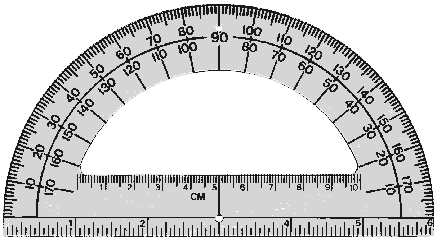Protractor

A tool used to measure angles.

8

*

Degree

The unit used to measure the size of an angel.

9Right Angle

An angel that measures 90* and forms a square corner.

10Acute Angle

An angle that measures less than 90* (cute!)

11Obtuse Angle

An angle that measures more than 90* (fat!)

12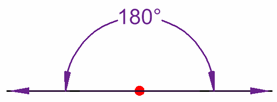Straight Angle

An angle that measures exactly 180* (line)

13Intersecting Lines

Lines that cross each other to form 4 angles.

14Parallel Lines

Lines in a plane that never intersect and are ALWAYS the same distance apart.

15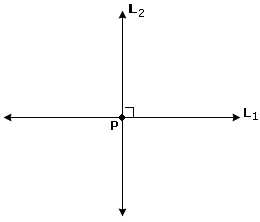Perpendicular Lines

Lines in a plane that meet and form a RIGHT angle.

16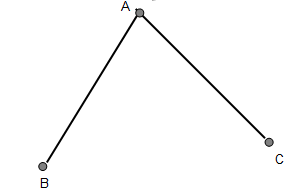Vertex

The point at which two rays meet to form an angle.

17Polygon

A closed plan figure with straight sides.

18Triangle

A polygon with three sides.

19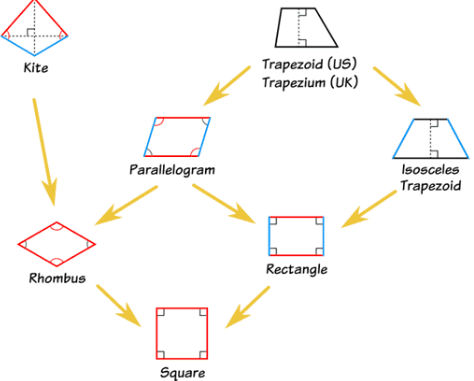A polygon with four sides.

20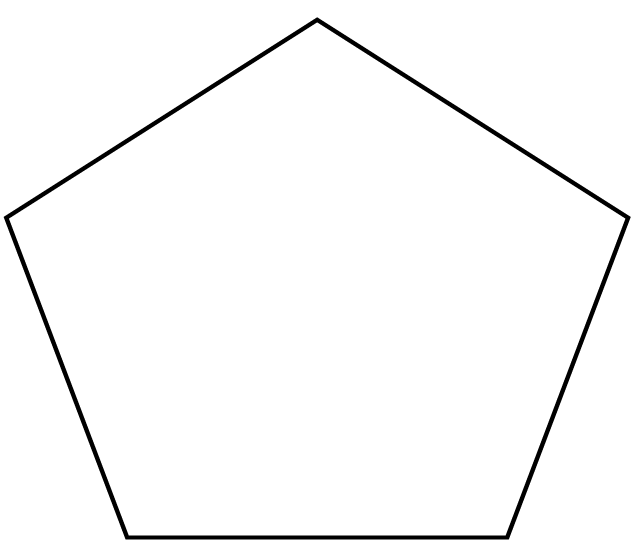Pentagon

A polygon with five sides.

21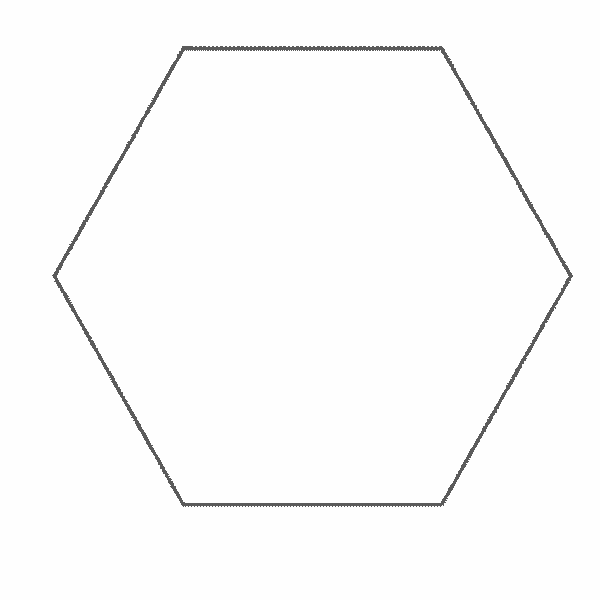Hexagon

A polygon with six sides.

22Octagon

A polygon with eight sides.

23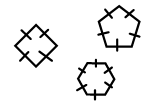Regular Polygon

A polygon where all sides and angles are equal.

24Irregular Polygon

A polygon where all angles and sides are not equal.

25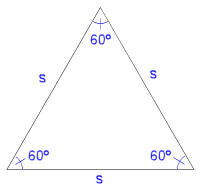Equilateral Triangle

A triangle with three equal sides.

26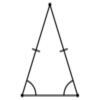Isosceles Triangle

A triangle with three equal sides.

27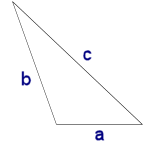Scalene Triangle

A triangle with no equal length sides.

28Right Triangle

A triangle with one right angle.

29Acute Triangle

A triangle with 3 acute angles.

30Obtuse Triangle

A triangle with 1 obtuse angle.

31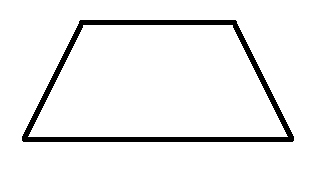Trapezoid

A quadrilateral with exactly one pair of parallel lines.

32Venn Diagram

A diagram that shows relationships (how things are the same and different) between things.

33Line Symmetry

What a figure has if it can be folded along a line so that both sides match perfectly.

34Rotational Symmetry

What a figure has if it can be turned around a central point and still look the same in two positions.

35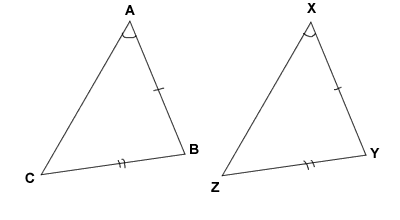Congruent

Having the same size and shape.

36Similar

Having the same shape but different size.

37

(7,6)

Ordered Pair

Two numbers that are used to locate a point on a coordinate grid.

38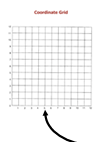X-Axis

The horizontal line on a coordinate grid. (like a bed)

39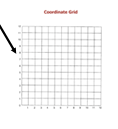Y-Axis

The vertical side of a coordinate grid. (Think, a pillar)

40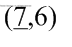X-Coordinate

The first number in an ordered pair.

41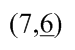Y-Coordinate

The second number in an ordered pair.

42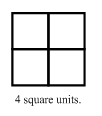Area

The space inside of a shape. It is measured in square units. The formula is a= l x w (area = length x width)

43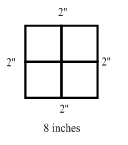Perimeter

The the sum of all of the sides.

44Cone

a solid, pointed figure that has a flat round base.

45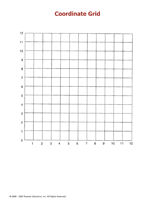Coordinate Grid

A grid formed by a horizontal line called the x-axis and a vertical line called the y-axis.

46Cube

A solid figure with six congruent square faces

47Cylinder

A solid figure that is shaped like a can.

48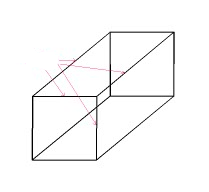Edge

The line segment where two or more faces of a solid figure meet.

49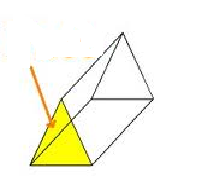Face

A polygon that is the flat surface of a solid figure.

50Net

A two-dimensional (flat) pattern that can be foled to make a three-dimensional figure.

51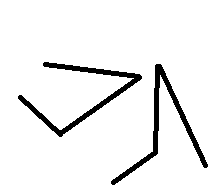Open figure

A figure that does not begin and end at the same point.

52Square Pyramid

A solid figure with a square base and four triangular sides that meet at a single point.

53Rectangular Prism

A solid figure in which all six faces are rectangles.

54Sphere

A round object whose curved surface is the same distance from the center to all its points.

55Triangular Prism

A solid figure that has two triangular bases and three rectangular bases.